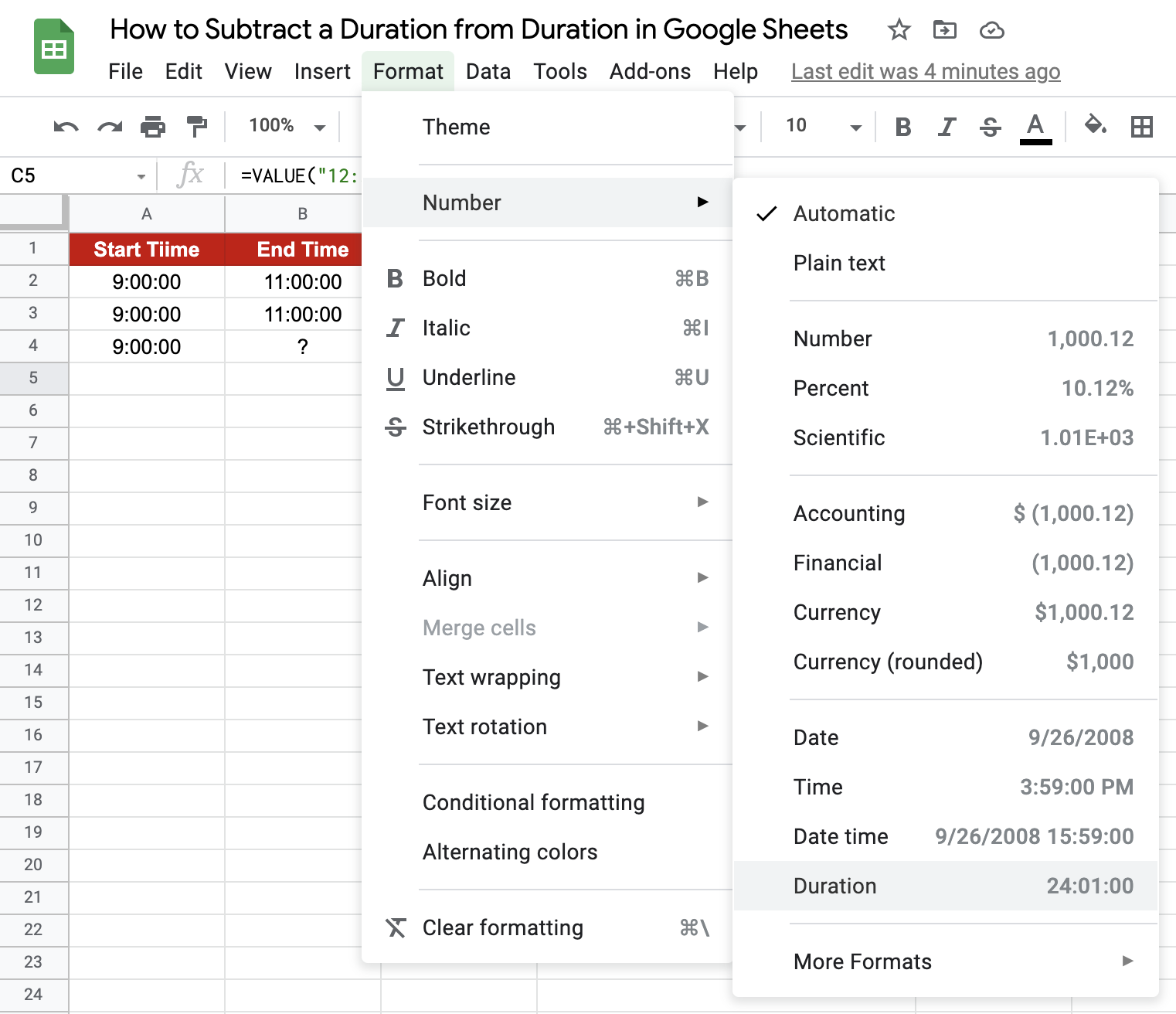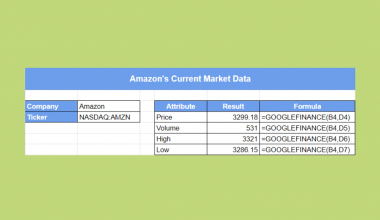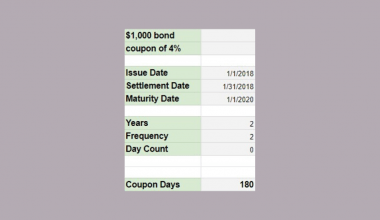# How to Subtract a Duration from Duration in Google Sheets

Often, while working with durations in Google Sheets, you find the need to obtain the difference between two durations to either be used for further calculations or just a simple representation of the same. In this guide, we will go through some functions and formats in Google Sheets that will enable you to seamlessly do this.

What is a duration? A duration represents a time period as determined by hours, minutes or seconds, typically in the HH:MM:SS.

This is not to be confused with the in-built Google Sheet function `DURATION()` which is a more specific time period denoting the number of compounding periods required for a monetary investment of a given present value to be appreciated at a given rate of interest to reach a target value.

Let us take a closer look at ways to get this difference (in durations), with examples.

## How to Get a Difference in Duration

### Straightforward subtraction

When you have the two durations as entries in two cells of a Google Sheet, the difference may be obtained by a simple formula as shown below:The term ‘Start Time’ refers to the initial duration, whereas ‘End Time’ refers to the final duration, the difference of which is of interest to us. We can get the desired result in two ways, both of which are essentially just subtracting one value from the other:

• Duration = B2 – A2
• Duration = MINUS(B3,A3)

### Subtract hard-coded values

What about when you want to hard-code either of the values into the formula? Assume you know the ‘Start Time’ is always going to be 9 a.m. (represented by 9:00:00 in the HH: MM: SS format), and you want to find the time elapsed at various points throughout the day. You could then try something like this:Duration = VALUE(“12:30:00”) – A4

You will get the output as shown below.  The `VALUE()` function here converts a given string into a number in any of the date, time, or number formats supported by Google Sheets.A couple of things to note here:

1. If you use the `TIMEVALUE()` function instead of the `VALUE()` function, you will get the same result, but you may get the wrong answer at times. And therefore, it is safer to use the `VALUE()` function.
2. You can hard-code both durations in the formula by using the `VALUE()` function, as shown above for the ‘End Time’.

When you use the `VALUE()` function to hard-code both durations, you will notice that the output is not in the HH: MM: SS format, but rather a numeric value. In this case, when we subtract two hard-coded durations, we get 0.0625:What does this number mean? This number simply means time as denoted by the ‘fraction of a day’ value. For example, 0.0625 represents 6.25% of a day, which is equal to 1.5 hours, which is the actual difference in duration based on the hard-coded values. To convert this numeric value into the more recognized format of HH:MM::SS, click on the cell (C5 in this case), go to the Format tab and navigate to the duration format:This will convert the numeric value to the required duration format:## How to Handle a “Negative” Duration

Another important thing to note while dealing with duration would be to deal with negative values as the difference between two durations. Let us say we want to get the difference between the two durations as given below:

Duration = VALUE(“10:30:00”) – VALUE(“12:00:00”)

The function will not throw an error and will output a numeric value of -0.0625. This is because the `VALUE()` function’s output is in numeric format by default, and thus subtraction is permitted. But a negative duration doesn’t really make sense now, does it?

There are a couple of ways to deal with this, making use of other formulas in Google Sheets. I’ll list a couple of them and explain why.

• Using `IF()`: Using the built-in function `IF()`, we can assign either a message that says “The End Time value must be greater than the Start Time value” or a hard-coded value of 0 for cases where the difference is negative. The same formula as above may be rewritten thus:

Duration = IF(VALUE(“10:30:00”) – VALUE(“12:00:00”) > 0, VALUE(“10:30:00”) – VALUE(“12:00:00”), 0)

• Using `MAX()`: A more efficient method of obtaining the above result would be to use the `MAX()` function and compare the output to 0:

Duration = MAX(0,VALUE(“10:30:00”) – VALUE(“12:00:00”))

Refer to our article on how to use IF () functions in Google Sheets for a more in-depth explanation.

## How to Represent a Difference in Duration

There are many different ways that you can represent a duration value (or a difference of duration values) in Google Sheets. We will go through the more popular ones among them, and you may use the one that is most suited to your needs.

### Duration Without the Seconds

The default format for representing a number as a duration in Google Sheets (as obtained through Format -> Number -> Duration) is:.

• 1 is formatted as 24:00:00 (since it represents 1 day, which is 24 hours).
• 1.2 is formatted as 28:48:00
• 0.03125 is formatted as 0:45:00.

If you need the format to omit the seconds, say, it makes your cell really long, and you want a cleaner way of representing the duration. There is a way to achieve this in Google Sheets. Navigate to Format -> Number -> More Formats -> Custom number format.Once you are on the Custom Number Formatting screen, enter [h]:mm and click Apply.You will get the output in the desired format:

You can also use the `TEXT()` function to get the same result as shown below:Note: Why can’t I get the same result by setting the cell’s Date/Time format explicitly?

That is because a value of say 1.5 will be represented as 12:00 and not as 36:00 (which would be the desired result).

### Difference of Durations in Hours, Minutes, and Seconds

You can represent a duration (or a difference between two durations) with one time unit, disregarding all the other units. For example, you may represent it as a measure of (only) hours, (only) minutes or (only) seconds. I will show you a couple of ways to do this.

One obvious way to get the desired result is to simply make use of the `HOUR()`, `MINUTE()` and `SECOND()` functions on the difference.You will get the output as below:Another way to achieve the same result without using any formulas is to just subtract durations first, and then apply the elapsed time format from Format -> Number -> More Formats -> More date and time formats. If you click the down arrow to the right of the text field, you’ll be able to choose between additional date and time units:You will get the same output as if you used the `HOUR()`, `MINUTE()` and `SECOND()` formulas. We have a comprehensive guide on how to convert seconds back to the HH:MM:SS format in one of our articles here. Feel free to go through and try converting what you converted into seconds back to the original duration format.

That’s pretty much it. You have everything you need to get started with durations on Google Sheets. You may make a copy of the spreadsheet using the link I have attached below:

Experiment with it using the other numerous Google Sheets formulas to enhance the functionality of dealing with durations.Our goal this year is to create lots of rich, bite-sized tutorials for Google Sheets users like you. If you liked this one, you'll love what we are working on! Readers receive ✨ early access ✨ to new content.

##### You May Also LikeThe GOOGLEFINANCE function in Google Sheets is useful when you need to retrieve current or historical market data…## How to Fix ‘Circular Dependency Detected’ Error in Google Sheets

The circular dependency detected errors in Google Sheets is due to the formula being within the range you’re…## How to Count Rows Between Two Values in Google Sheets

Knowing how to count rows between two values in Google Sheets is useful if you want to find…## How to Use MAXIFS Function in Google Sheets

The MAXIFS function in Google Sheets is useful to return the maximum value of a range based on…